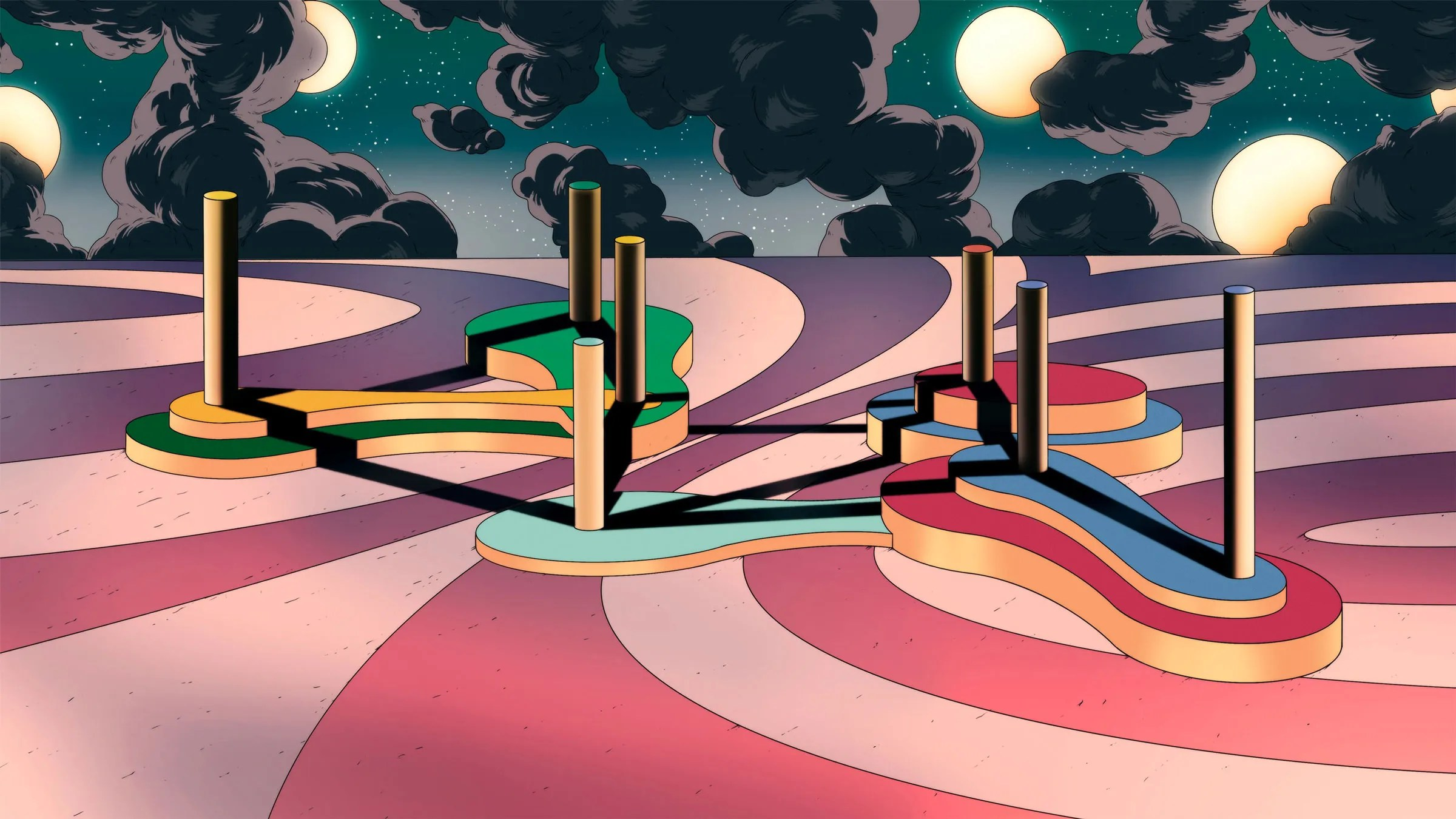# How Big Data Carried Graph Theory Into New Dimensions

Graph theory isn’t enough.

The mathematical language for talking about connections, which usually depends on networks—vertices (dots) and edges (lines connecting them)—has been an invaluable way to model real-world phenomena since at least the 18th century. But a few decades ago, the emergence of giant data sets forced researchers to expand their toolboxes and, at the same time, gave them sprawling sandboxes in which to apply new mathematical insights. Since then, said Josh Grochow, a computer scientist at the University of Colorado, Boulder, there’s been an exciting period of rapid growth as researchers have developed new kinds of network models that can find complex structures and signals in the noise of big data.

Grochow is among a growing chorus of researchers who point out that when it comes to finding connections in big data, graph theory has its limits. A graph represents every relationship as a dyad, or pairwise interaction. However, many complex systems can’t be represented by binary connections alone. Recent progress in the field shows how to move forward.

Consider trying to forge a network model of parenting. Clearly, each parent has a connection to a child, but the parenting relationship isn’t just the sum of the two links, as graph theory might model it. The same goes for trying to model a phenomenon like peer pressure.

“There are many intuitive models. The peer pressure effect on social dynamics is only captured if you already have groups in your data,” said Leonie Neuhäuser of RWTH Aachen University in Germany. But binary networks don’t capture group influences.

Mathematicians and computer scientists use the term “higher-order interactions” to describe these complex ways that group dynamics, rather than binary links, can influence individual behaviors. These mathematical phenomena appear in everything from entanglement interactions in quantum mechanics to the trajectory of a disease spreading through a population. If a pharmacologist wanted to model drug interactions, for example, graph theory might show how two drugs respond to each other—but what about three? Or four?

While the tools for exploring these interactions are not new, it’s only in recent years that high-dimensional data sets have become an engine for discovery, giving mathematicians and network theorists new ideas. These efforts have yielded interesting results about the limits of graphs and the possibilities of scaling up.

“Now we know that the network is just the shadow of the thing,” Grochow said. If a data set has a complex underlying structure, then modeling it as a graph may reveal only a limited projection of the whole story.Emilie Purvine of the Pacific Northwest National Laboratory is excited about the power of tools like hypergraphs to map out subtler connections between data points. Photograph: Andrea Starr/Pacific Northwest National Laboratory

“We’ve realized that the data structures we’ve used to study things, from a mathematical perspective, aren’t quite fitting what we’re seeing in the data,” said the mathematician Emilie Purvine of the Pacific Northwest National Laboratory.

Which is why mathematicians, computer scientists, and other researchers are increasingly focusing on ways to generalize graph theory—in its many guises—to explore higher-order phenomena. The last few years have brought a torrent of proposed ways to characterize these interactions, and to mathematically verify them in high-dimensional data sets.

For Purvine, the mathematical exploration of higher-order interactions is like the mapping of new dimensions. “Think about a graph as a foundation on a two-dimensional plot of land,” she said. The three-dimensional buildings that can go on top could vary significantly. “When you’re down at ground level, they look the same, but what you construct on top is different.”

Enter the Hypergraph

The search for those higher-dimensional structures is where the math turns especially murky—and interesting. The higher-order analogue of a graph, for example, is called a hypergraph, and instead of edges, it has “hyperedges.” These can connect multiple nodes, which means it can represent multi-way (or multilinear) relationships. Instead of a line, a hyperedge might be seen as a surface, like a tarp staked in three or more places.

Which is fine, but there’s still a lot we don’t know about how these structures relate to their conventional counterparts. Mathematicians are currently learning which rules of graph theory also apply for higher-order interactions, suggesting new areas of exploration.

To illustrate the kinds of relationship that a hypergraph can tease out of a big data set—and an ordinary graph can’t—Purvine points to a simple example close to home, the world of scientific publication. Imagine two data sets, each containing papers coauthored by up to three mathematicians; for simplicity, let’s name them A, B, and C. One data set contains six papers, with two papers by each of the three distinct pairs (AB, AC, and BC). The other contains only two papers total, each coauthored by all three mathematicians (ABC).